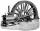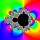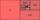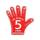Computer

The computer was purchased 10000,-. Each year, the price of a computer depreciates always the same percentage of the previous year. After four years, the value of the computer is reduced to 1300,- How many percent was depreciated price of the computer each year?

Result

p =  39.95 %

Solution:Leave us a comment of example and its solution (i.e. if it is still somewhat unclear...):Be the first to comment!Next similar examples:

1. BankPaul put 10000 in the bank for 6 years. Calculate how much you will have in the bank if he not pick earned interest or change deposit conditions. The annual interest rate is 3.5%, and the tax on interest is 10%.
2. MachinePrice of the new machine is € 62000. Every year is depreciated 15% of residual value. What will be the value of the machine after 3 years?
3. Five membersWrite first 5 members geometric sequence and determine whether it is increasing or decreasing: a1 = 3 q = -2
4. CalculationHow much is sum of square root of six and the square root of 225?
5. GP - 8 itemsDetermine the first eight members of a geometric progression if a9=512, q=2
6. Tenth memberCalculate the tenth member of geometric sequence when given: a1=1/2 and q=2
7. Geometric progression 48,4√2,4,2√2
8. Theorem proveWe want to prove the sentence: If the natural number n is divisible by six, then n is divisible by three. From what assumption we started?
9. Geometric sequence 4It is given geometric sequence a3 = 7 and a12 = 3. Calculate s23 (= sum of the first 23 members of the sequence).
10. Six termsFind the first six terms of the sequence a1 = -3, an = 2 * an-1
11. Geometric progression 2There is geometric sequence with a1=5.7 and quotient q=-2.5. Calculate a17.
12. Geometric progressionFill 4 numbers between 4 and -12500 to form geometric progression.
13. PowerNumber ?. Find the value of x.
14. SequenceFind the common ratio of the sequence -3, -1.5, -0.75, -0.375, -0.1875. Ratio write as decimal number rounded to tenth.
15. A perineumA perineum string is 10% shorter than its original string. The first string is 24, what is the 9th string or term?
16. Gp - 80Sum of the first four members of a geometric progression is 80. Determine they if we know that the fourth member is nine times greater than the second.
17. ValueFind the value of the expression: 6!·10^-3